Ex 14.1

Chapter 14 Class 8 Factorisation
Serial order wise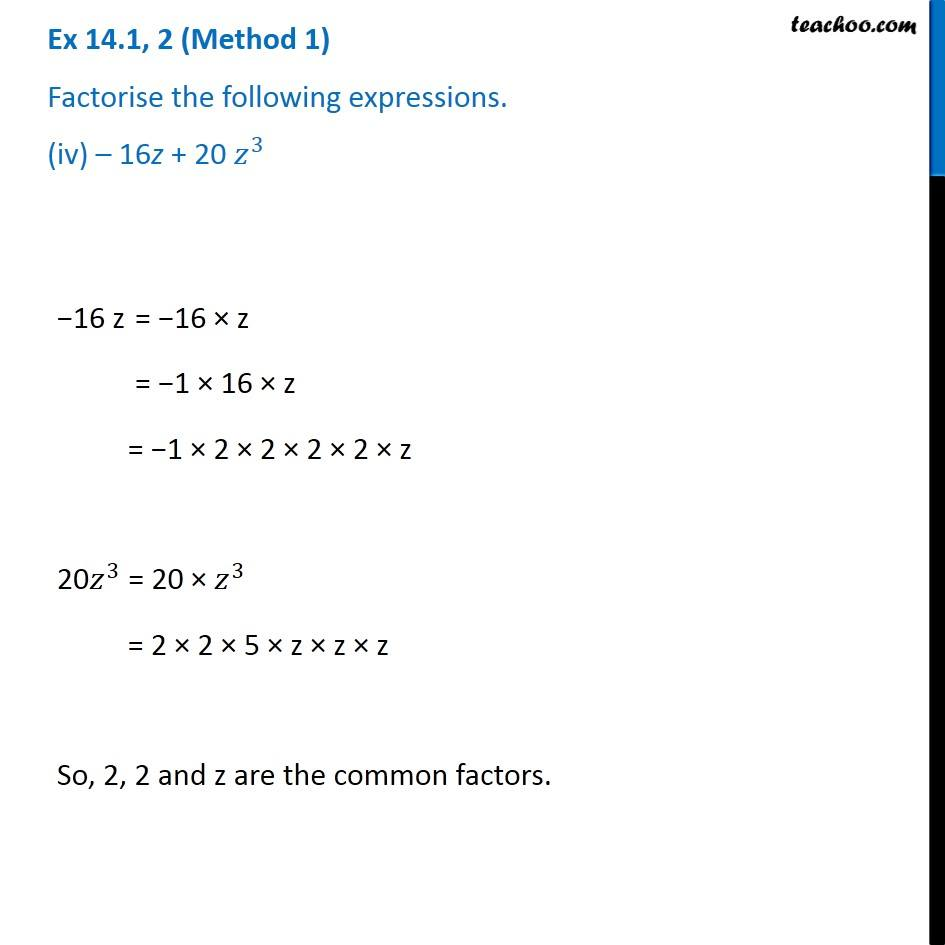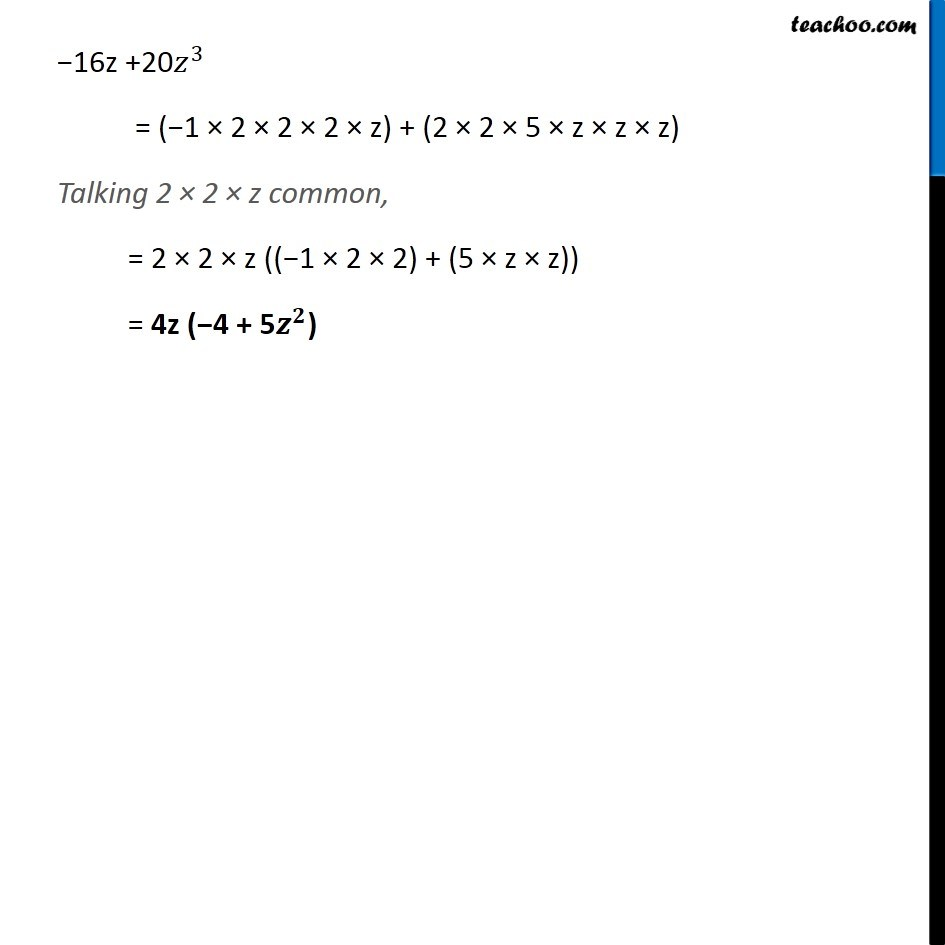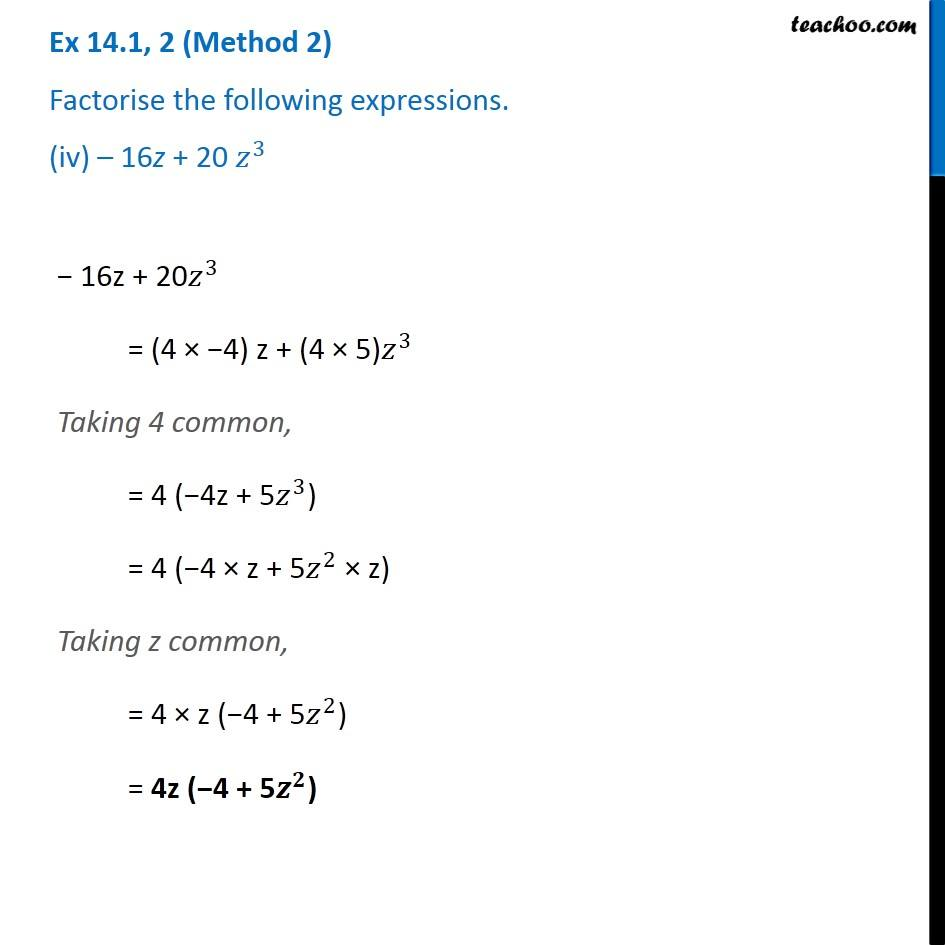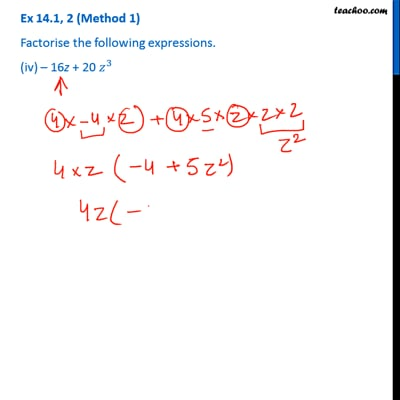This video is only available for Teachoo black users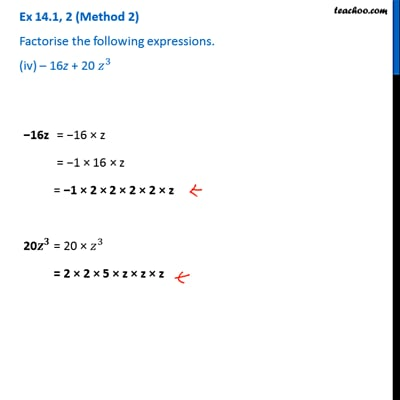This video is only available for Teachoo black users

Get live Maths 1-on-1 Classs - Class 6 to 12

### Transcript

Ex 14.1, 2 (Method 1) Factorise the following expressions. (iv) – 16z + 20 𝑧^3 −16 z = −16 × z = −1 × 16 × z = −1 × 2 × 2 × 2 × 2 × z 20𝑧^3 = 20 × 𝑧^3 = 2 × 2 × 5 × z × z × z So, 2, 2 and z are the common factors. −16z +20𝑧^3 = (−1 × 2 × 2 × 2 × z) + (2 × 2 × 5 × z × z × z) Talking 2 × 2 × z common, = 2 × 2 × z ((−1 × 2 × 2) + (5 × z × z)) = 4z (−4 + 5𝒛^𝟐) Ex 14.1, 2 (Method 2) Factorise the following expressions. (iv) – 16z + 20 𝑧^3 − 16z + 20𝑧^3 = (4 × −4) z + (4 × 5)𝑧^3 Taking 4 common, = 4 (−4z + 5𝑧^3) = 4 (−4 × z + 5𝑧^2 × z) Taking z common, = 4 × z (−4 + 5𝑧^2) = 4z (−4 + 5𝒛^𝟐)